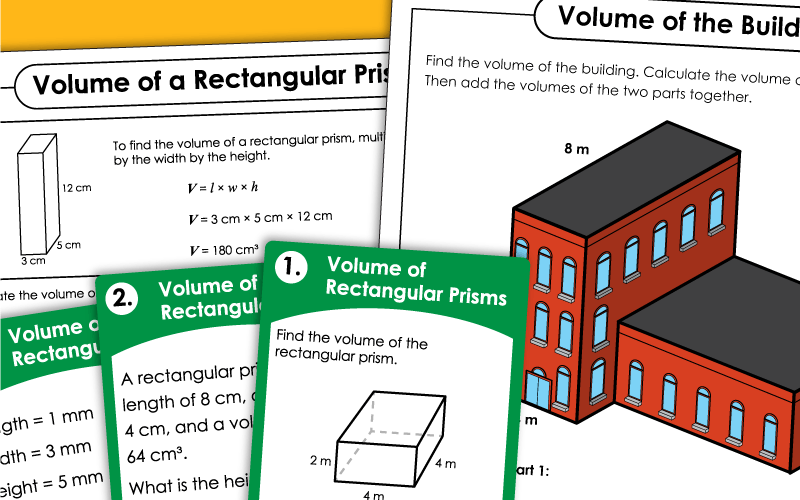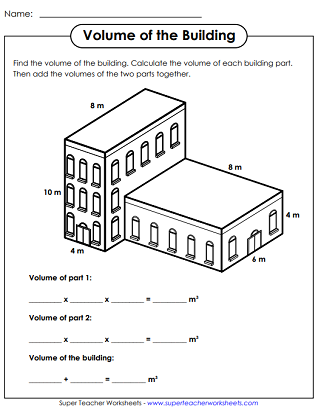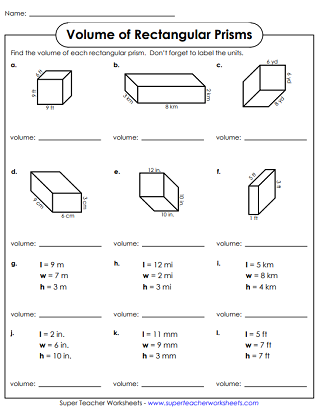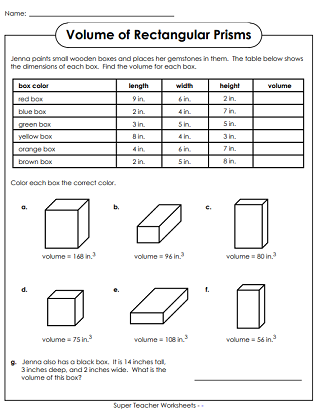# Volume of a Rectangular Prism

On these activities, students will calculate the volumes of the rectangular prisms, using the formula: Volume = length x width x height.## Volume of Rectangular Prisms (Basic: Whole Numbers)

Multiply the length times width times height to determine the volume of each rectangular prism.
On this worksheet, students must use data in the table to calculate volume.  Then, they color the boxes the correct colors according to their answers.
This file contains 30 task cards. Find the volumes of each rectangular prism.
Students multiply three numbers together to find the volume of each rectangular prism shape.
This volume worksheet includes 4 types of problems.  There are rectangular prisms drawn with cubes and drawn with dimensions.  There is also a word problem.
Calculate the volume of this moving van to find out how much space the family has to fit everything in it.
Find the volume of the planter to figure out how much soil is needed to fill it.

## Triangular Prisms

Calculate the volumes of the triangular prisms.

## Volume of Rectangular Prisms (Advanced: Decimals)

Students use the formula for finding volume of a rectangular prism. This worksheet uses decimals in one, two, or all three dimensions.
Using the table, students find the volume of various boxes and color the illustrations based on the results. Decimals are used in the measurements of the sides.
Multiply length times width times height to find the volumes of the 30 rectangular prisms on these task cards. Advanced level includes one or more decimal measurements on each shape.
Students find the volume of 12 rectangular prisms with decimal dimension measurements.
Volume of Composite Figures

Find the volumes of pairs of connected, non-overlapping rectangular prisms.

More Volume Worksheets

Find printable worksheets for teaching volume of cylinders, cones, spheres, prisms, and more.

## Volume Worksheet ImagesMy Account
Site Information